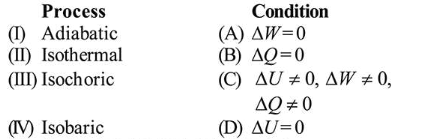# Match the thermodynamic processes taking place in a system with the correct conditions. In the table :

Question:

Match the thermodynamic processes taking place in a system with the correct conditions. In the table : $\Delta Q$ is the heat supplied, $\Delta W$ is the work done and $\Delta U$ is change in internal energy of the system.1. (I)-(A), (II)-(B), (III)-(D), (IV)-(D)

2. (I)-(B), (II)-(A), (III)-(D), (IV)-(C)

3. (I)-(A), (II)-(A), (III)-(B), (IV)-(C)

4. (I)-(B), (II)-(D), (III)-(A), (IV)-(C)

Correct Option: , 4

Solution:

(4)

(I) Adiabatic process : No exchange of heat takes place with surroundings.

$\Rightarrow \Delta Q=0$

(II) Isothermal process : Temperature remains constant

$\therefore \Delta T=0 \Rightarrow \Delta U=\frac{f}{2} n R \Delta T \Rightarrow \Delta U=0$

No change in internal energy $[\Delta U=0]$.

(III) Isochoric process volume remains constant

$\Delta V=0 \Rightarrow W=\int P \cdot d V=0$

Hence work done is zero.

(IV) In isobaric process pressure remains constant.

$W=P \cdot \Delta V \neq 0$

$\Delta U=\frac{f}{2} n R \Delta T=\frac{f}{2}[P \Delta V] \neq 0$

$\therefore \Delta Q=n C_{p} \Delta T \neq 0$